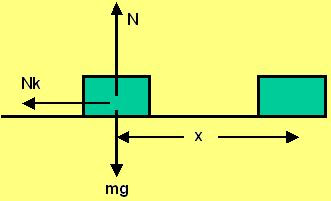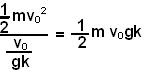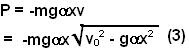## Friday, May 2, 2008

### Irodov Problem 1.127Say the mass acquires a velocity v0 at time t=0, the friction force Nk acts in the direction opposite to the direction of motion and hence decelerates it, N being the normal reaction from the surface. Along the vertical direction there are two forces acting on the mass, the normal reaction N and the force of gravity mg. There is no acceleration in the vertical direction and so we have,

N-mg=0 (1)

The mass hence experiences a deceleration Nk/m at any instant of time. The work done by friction at any instant is -Nkv, where v is the instantaneous horizontal velocity of the mass. The negative sign appears since the direction of motion of the mass is exactly in the opposite direction to the force of friction.

a) In this part the force of friction is constant given by Nk = mgk and hence experiences a constant deceleration of -gk. The time taken for the block to come to complete rest is v0/gk.
Initially the mass had a kinetic energy ofand due to friction it will loose all of it when it stops. The total negative work done by friction will then be equal to the initial kinetic energy of the mass i.e.. The average power generated by friction is total work done by total time given by,b) In this part the force of friction depends on distance from initial position as. Thus, as any given time,The instantaneous power generated by friction is given by -mgkv and is thus given by,The extrema (maxima or minima) occurs whenFrom (4) and (3) we have,